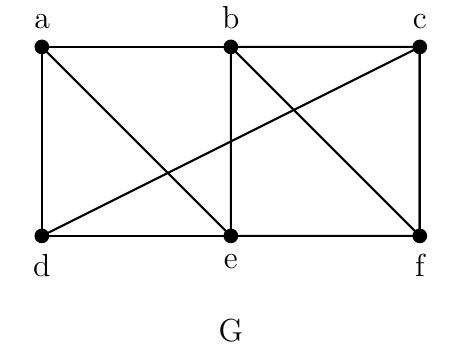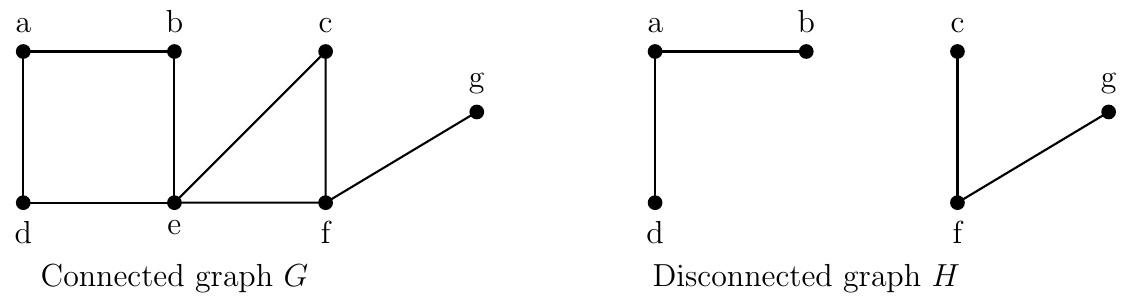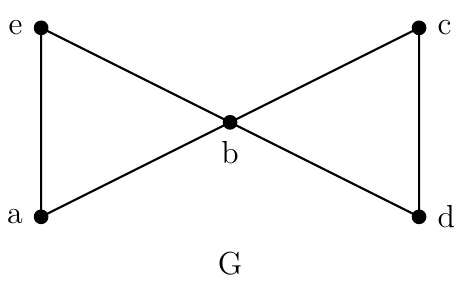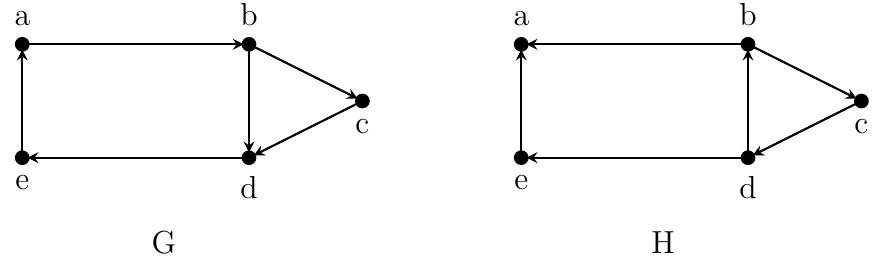Discrete Math Home

## Connectivity

Definition (Walk). A walk of length $$n$$ from vertex $$v_0$$ to $$v_n$$ in a graph $$G$$ is a sequence of vertices and edges of the form $v_0,e_1,v_1,e_2,v_2,\ldots,v_{n-1},e_n,v_n$ where edge $$e_i$$ is an edge between vertices $$v_{i-1}$$ and $$v_i$$ for all $$i=1,2,\ldots,n$$. A circuit or a closed walk is a walk whose endpoints are the same (i.e., $$v_0=v_n$$).

Remark. A walk may repeat a vertex and an edge. A path of length $$n$$ between vertices $$v_0$$ to $$v_n$$ is a walk of length $$n$$ from $$v_0$$ to $$v_n$$ that has no repeated vertices and edges which can be simply written in the form $$v_0v_1v_2\cdots v_n$$ in a simple graph.Example. In the above graph $$G$$, $$abcf$$ is a walk and also a path of length $$3$$. $$abcfb$$ is a simple walk of length 4, but not a path because of the repeated vertex $$b$$. The walk $$abcfba$$ is a circuit of length 6.

Definition (Distance). The distance between vertices $$u$$ to $$v$$ in a graph $$G$$, denoted by $$\operatorname{d}(u,v)$$, is the minimum length of a path (or a walk) between $$u$$ and $$v$$. If there is no path between $$u$$ and $$v$$, we define $$\operatorname{d}(u,v)=\infty$$.

Example. In the above graph $$G$$, $$\operatorname{d}(a,a)=0$$, $$\operatorname{d}(a,e)=1$$, and $$\operatorname{d}(a,f)=2$$.

Remark. The concept of distance is used to define Erdös number (the collaboration distance from Paul Erdös), Bacon number etc. In 2016, the average distance in the Facebook friend graph was $$4.57$$, i.e., $$3.57$$ intermediaries or "degrees of separation." It is said that everyone on the planet is connected to everyone else by six other people ("six degrees of separation").

Definition (Connected graph). A graph $$G$$ is connected if there is a path between any two vertices of $$G$$. $$K_1$$ is defined to be connected. A graph is disconnected if it is not connected. A connected component or simply component of a graph $$G$$ is a connected subgraph of $$G$$ that is not a proper subgraph of another connected subgraph of $$G$$.Example. The above graph $$G$$ is connected, i.e., it has one connected component. But the above disconnected graph $$H$$ has two connected components.

Example. $$99.91\%$$ of Facebook users belong to a single large connected component in the Facebook friend graph.

Definition (Cut vertex). A vertex $$v$$ is a cut vertex of a graph $$G$$ if $$G-v$$ has more connected components than $$G$$. An edge $$e$$ is a cut edge or bridge of a graph $$G$$ if $$G-e$$ has more connected components than $$G$$.

Example. The vertex $$e$$ is a cut vertex of the above graph $$G$$. Note that if $$v$$ is a cut vertex of a connected graph $$G$$, then $$G-v$$ is disconnected. The edge $$\{f,g\}$$ is a bridge of both $$G$$ and $$H$$. Note that $$K_n,\;n\geq 3$$ has no cut vertices and bridges.

Definition (Edge connectivity). For a connected graph $$G=(V,E)$$, an edge cut of $$G$$ is a subset $$E'$$ of $$E$$ for which $$G-E'$$ is disconnected. The edge connectivity of $$G$$, denoted by $$\lambda(G)$$, is the minimum number of edges in an edge cut of $$G$$. We define $$\lambda(G)=0$$ for disconnected graphs $$G$$ and for $$G=K_1$$.

Remark. Note that $$\lambda(G)=n-1$$ if and only if $$G=K_n$$. So $$0\leq \lambda(G)\leq n-1$$ for any graph $$G$$ on $$n$$ vertices.

Example. $$\lambda(P_n)=1$$ for $$n\geq 2$$ and $$\lambda(C_n)=2$$.

Definition (Vertex connectivity). For a connected graph $$G=(V,E)$$, a vertex cut or separating set of $$G$$ is a subset $$V'$$ of $$V$$ for which $$G-V'$$ is disconnected. The vertex connectivity of $$G$$, denoted by $$\kappa(G)$$, is the minimum number of vertices in a vertex cut of $$G$$. Since $$K_n$$ has no vertex cut, we define $$\kappa(K_n)=n-1$$. We define $$\kappa(G)=0$$ for disconnected graphs $$G$$. A graph $$G$$ is $$k$$-connected if $$\kappa(G)\geq k$$.

Remark. $$0\leq \kappa(G)\leq n-1$$ for any graph $$G$$ on $$n$$ vertices. Note that if $$G$$ is $$k$$-connected, then $$G$$ is $$j$$-connected for all $$j=0,1,\ldots,k$$. Also $$\kappa(G)$$ is the greatest integer $$k$$ for which $$G$$ is $$k$$-connected.

Example.

1. $$\kappa(G)\geq 1$$ for any connected graph $$G$$. So a connected graph is $$1$$-connected.

2. $$\kappa(C_n)=2$$. So $$C_n$$ is $$2$$-connected.

The minimum degree of a vertex in a graph $$G$$ is denoted by $$\delta(G)$$.

Theorem (Whitney, 1932). For a simple graph $$G$$, $$\kappa(G)\leq\lambda(G)\leq \delta(G)$$.Example. Consider the above graph $$G$$.

1. Find the vertex cuts and edge cuts of $$G$$ of size at most $$2$$.

2. Find $$\kappa(G)$$, $$\lambda(G)$$, and $$\delta(G)$$ to verify $$\kappa(G)\leq\lambda(G)\leq \delta(G)$$.

Solution. (a) The vertex cuts of $$G$$ of size at most $$2$$ are $$\{b\},\{b,a\},\{b,c\},\{b,d\},\{b,e\}$$. The edge cuts of $$G$$ of size at most $$2$$ are $$\{\{b,c\},\{b,d\}\}, \{\{b,a\},\{b,e\}\}$$, and the sets of two edges incident with $$a,e,c,d$$.

(b) $$\kappa(G)=1$$, $$\lambda(G)=2$$, $$\delta(G)=2$$, and we have $\kappa(G)=1 < 2=\lambda(G)=\delta(G).$

Connectivity of digraphs

Definition (Walk). A walk of length $$n$$ from vertex $$v_0$$ to $$v_n$$ in a digraph $$G$$ is a sequence of vertices and arcs of the form $v_0,e_1,v_1,e_2,v_2,\ldots,v_{n-1},e_n,v_n$ where arc $$e_i$$ is an arc from $$v_{i-1}$$ to $$v_i$$ for all $$i=1,2,\ldots,n$$. A circuit or a closed walk is a walk whose endpoints are the same (i.e., $$v_0=v_n$$).

Remark. A walk may repeat a vertex and an arc. A directed path of length $$n$$ from $$v_0$$ to $$v_n$$ is a walk of length $$n$$ from $$v_0$$ to $$v_n$$ that has no repeated vertices and arcs which can be simply written in the form $$v_0v_1v_2\cdots v_n$$ in a simple digraph.

Definition (Strongly connected digraph). A digraph $$G$$ is strongly connected if there is a walk from $$u$$ to $$v$$ and a walk from $$v$$ to $$u$$ for any two vertices $$u$$ and $$v$$. A directed graph $$G$$ is weakly connected if there is a walk between any two vertices in the underlying undirected graph of $$G$$.

Remark. If a digraph $$G$$ is strongly connected, then it is weakly connected. The converse is not true in general.Example. Above digraph $$G$$ is strongly connected and weakly connected. Above digraph $$H$$ is not strongly connected (since there is no walk from $$a$$ to $$b$$) but $$H$$ is weakly connected.

Definition (Strongly connected component). A subgraph of a digraph $$G$$ is called a strongly connected component if it is strongly connected and is not contained in a larger strongly connected subdigraph of $$G$$.

Example. The above digraph $$H$$ has 3 strongly connected components: $$a;e$$; and the subdigraph on vertices $$b,c,d$$ with arcs $$(b,c),(c,d),(d,b)$$.

Example. A webgraph in 1999 with 200 millions vertices and 1.5 billion arcs was studied. It was not strongly connected but it had lots of strongly connected components covering $$90\%$$ of the vertices of the webgraph.

Last edited